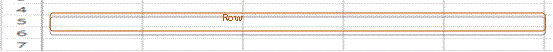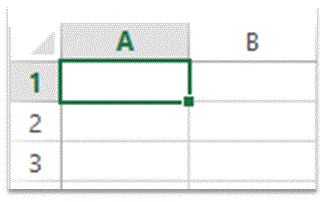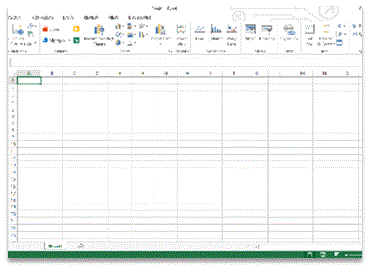# Important Terminologies in Microsoft Excel

## Important Terminologies in Microsoft Excel

Here you will find important terminologies that are used in Microsoft Excel. MS Excel is widely used all across the globe for its flexibility and high support for mathematical, statistical and financial calculations.

1.      What is meant by a Row?

A row is a horizontal block that refers to a single record. In MS Excel 2013, there are 1,048,576 rows in one Worksheet or Sheet which means it can hold this much of records in a single Sheet.2.      What is meant by a Column?

A column is a vertical block that refers to all records falling under one field. There are multiple column within a single row or record. In MS Excel 2013, there are 16,384 columns in one Worksheet or Sheet which means it can hold a record having this much fields in it.3.      What is meant by a Cell?

An intersection point, where a row is intersected by a column is called a Cell. This block contains the specific data residing in a particular record (row) under a particular field (column). The label for a cell contains the IDs of both the row and the column. For example, the point where Column A is intersected by Row 1, will be marked as Cell “A1” where “A” is taken from the column label and “1” is taken from the row label. This new label will be unique throughout the sheet and hence will be used for formulas and functions.4.      What is meant by a Worksheet?

A worksheet is a container that contains multiple rows and columns.5.      What is meant by a Workbook?

A workbook is a container that contains multiple worksheets.